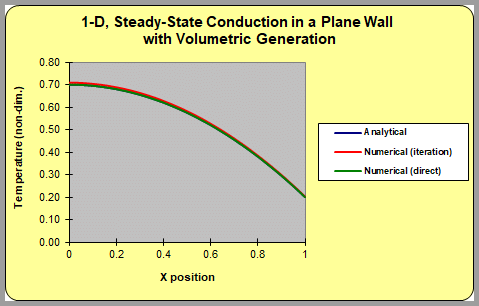# Solution of a Tridiagonal System of Linear Equations

UPDATED: 02/16/2021

This workbook solves a simple one-dimensional, steady-state conduction equation by finite-difference methods that results in a tridiagonal system of linear equations.  The left side of this plane wall is adiabatic by symmetry; the right side is exposed to convection.  This workbook demonstrates the solution of the coupled set of equations by a simple iteration scheme as well as by a direct solution of the tridiagonal system using an LAPACK routine.Temperature vs. distance in a plane wall corresponding to conduction with volumetric heat generation. The plots include: 1. the analytical solution, 2. a finite-difference solution where the equations are solved by iteration, and 3. finite-difference solution where the tridiagonal system of linear equations is solved directly by Gauss-elimination.